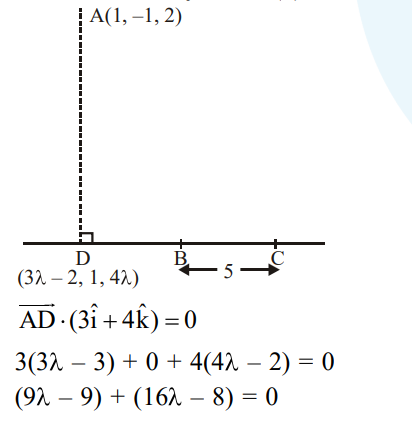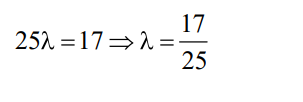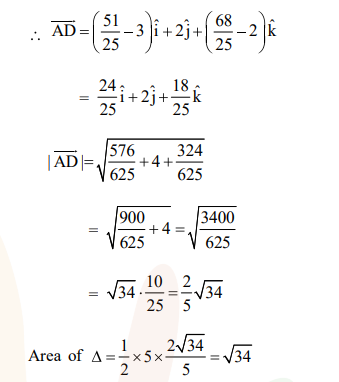# Solve this following`
Question:

The vertices $\mathrm{B}$ and $\mathrm{C}$ of a $\triangle \mathrm{ABC}$ lie on the line,

$\frac{x+2}{3}=\frac{y-1}{0}=\frac{z}{4}$ such that $B C=5$ units. Then the

area (in sq. units) of this triangle, given that the point $\mathrm{A}(1,-1,2)$, is :-

1. $2 \sqrt{34}$

2. $\sqrt{34}$

3. 6

4. $5 \sqrt{17}$

Correct Option: , 2

Solution: